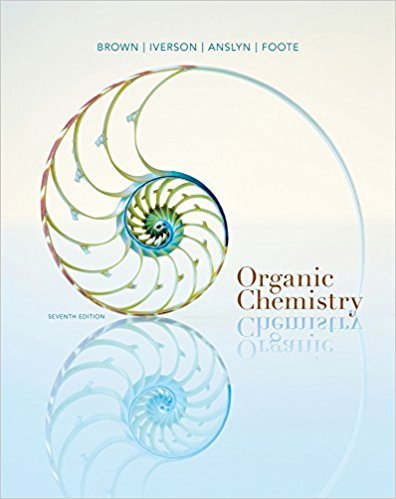×
Get Full Access to Organic Chemistry - 7 Edition - Chapter 22 - Problem 22.55
Get Full Access to Organic Chemistry - 7 Edition - Chapter 22 - Problem 22.55

×

# One potential synthesis of the anti-inflammatory and analgesic drug nabumetoneisISBN: 9781133952848 483

## Solution for problem 22.55 Chapter 22

Organic Chemistry | 7th Edition

• Textbook Solutions
• 2901 Step-by-step solutions solved by professors and subject experts
• Get 24/7 help from StudySoup virtual teaching assistantsOrganic Chemistry | 7th Edition

4 5 1 352 Reviews
30
2
Problem 22.55

One potential synthesis of the anti-inflammatory and analgesic drug nabumetoneis chloromethylation ( 22.48) of 2-methoxynaphthalene followed by anacetoacetic ester synthesis (Section 19.6). (a) Account for the regioselectivity of chloromethylation at carbon 6 rather than atcarbon 5 or 7.(b) Show steps in the acetoacetic ester synthesis by which the synthesis of nabumetoneis completed.

Step-by-Step Solution:
Step 1 of 3

I. Chapter 11 Continued A. Concentration Units 1. Molarity (M) a. M = moles/liters b. [KCl] means molarity of a KCl solution 2. Molality (m) a. m = moles/kg of solvent b. mass-based and independent of temperature 3. Mass Fraction a. Mass fraction = mass of solute/total mass b. Mass percent = mass fraction * 100% 4. Mass Percent a. Mass percent is parts per hundred i. Ppm = part per million ii. Ppb = parts per billion iii. Ppt = parts per trillion b. 1 ppm of solute in water = 1 mg/1000g of solution

Step 2 of 3

Step 3 of 3

##### ISBN: 9781133952848

Unlock Textbook Solution